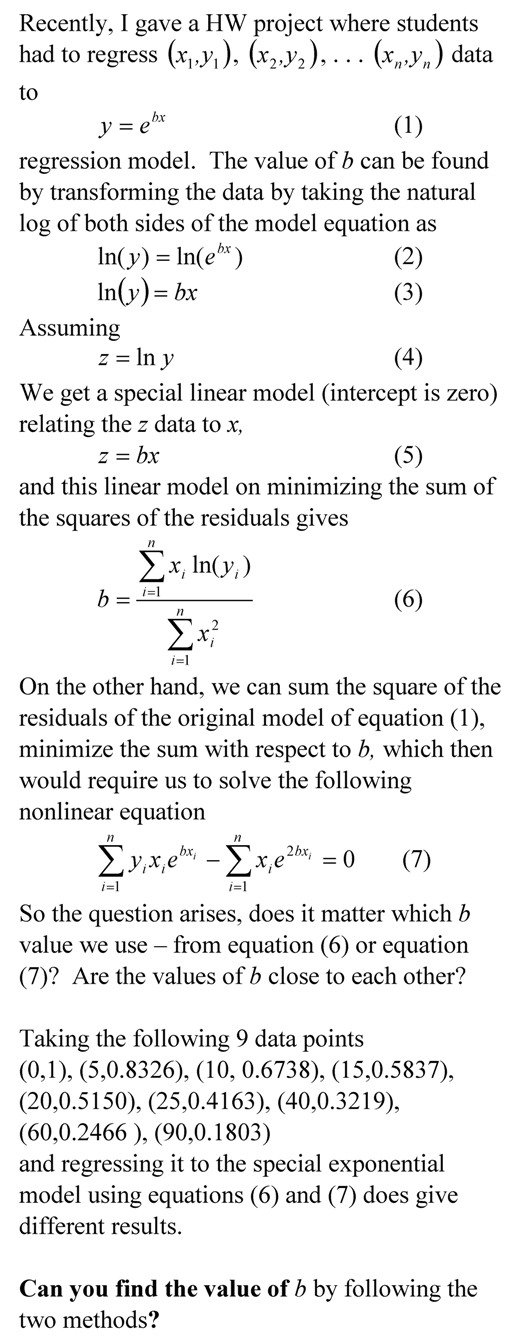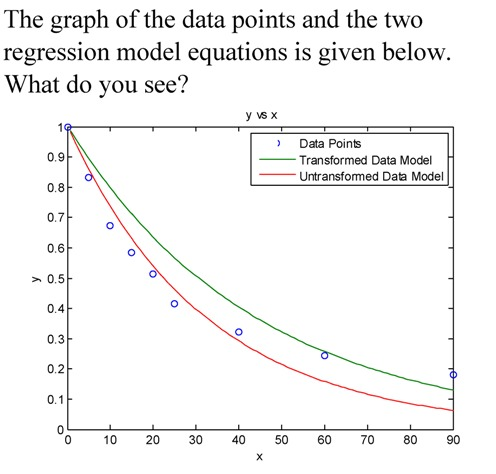## Does it make a large difference if we transform data for nonlinear regression models__________________________________________________

This post is brought to you by Holistic Numerical Methods: Numerical Methods for the STEM undergraduate at http://numericalmethods.eng.usf.edu, the textbook on Numerical Methods with Applications available from the lulu storefront, and the YouTube video lectures available at http://numericalmethods.eng.usf.edu/videos and http://www.youtube.com/numericalmethodsguy

## How do you know that the least squares regression line is unique and corresponds to a minimum

We already know that using the criterion of either

1. minimizing sum of residuals OR
2. minimizing sum of the absolute value of residuals

is BAD as either of the criteria do not give a unique line. Visit these notes for an example where these criteria are shown to be inadequate.

So we use minimizing the sum of the squares of the residuals as the criterion. How can we show that this criterion gives a unique line?

The proof is given below as image files because the proof is equation intensive. I made a better resolution pdf file also._____________________________________________________

This post is brought to you by Holistic Numerical Methods: Numerical Methods for the STEM undergraduate at http://numericalmethods.eng.usf.edu